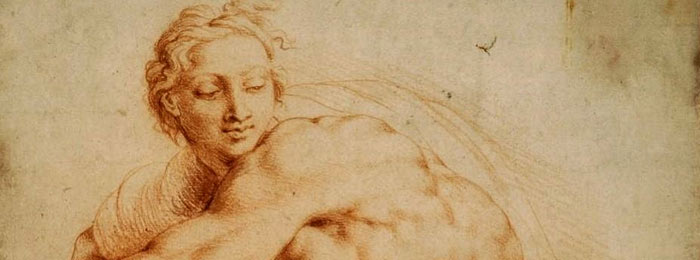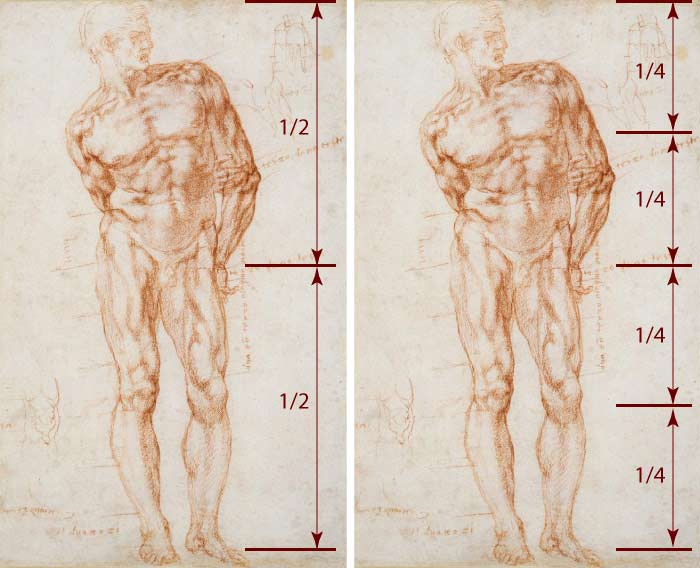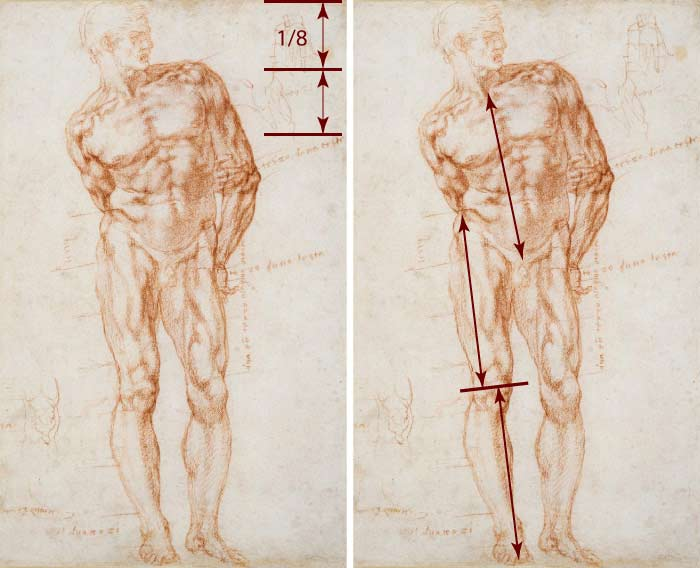## How to measure proportions of a human body?### Question from William, Anatomy Master Class student

When I attempt to do an “eight head figure,” my proportions are thrown off.

This one in particular:
“The distance between the toes and the top edge of the kneecap is the same as the distance from that top edge of the kneecap to the iliac crest. This is also the same as the distance from the pubis to the pit of the neck.”

I either make a figure with proportions longer than eight heads, or an eight head figure without proportions; I am confused.

How to measure proportions of a human body?

#### Feedback from Vladimir London, Anatomy Master Class tutor

Hi William,

Yes, you need to watch the video about figure proportions once again.

When you start drawing a standing figure, make sure you apply the following main proportions of the human body:

1. Divide the total height of the body in half – this would be at the level of the hip joints.

2. Divide both halves of the figure once again in half. The upper half-mark will be the middle of the chest. The lower one is the knee joint area.3. Divide the upper quarter in half; this would give you 1/8. Now, make the very top section a bit bigger—this would be the head, about 7 to 7.5 times of the height of the body.

4. It’s time to apply the proportion you so well quoted: “The distance between the toes and the top edge of the kneecap is the same as the distance from that top edge of the kneecap to the iliac crest. This is also the same as the distance from the pubis to the pit of the neck.”I hope this helps.

Best regards,